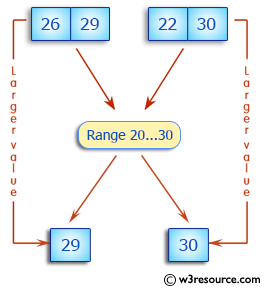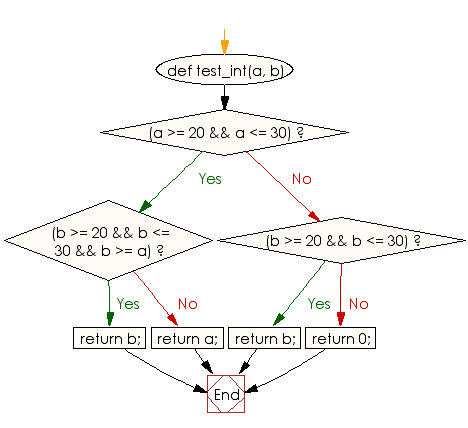﻿ Ruby Basic exercises: Check two positive integer values and return the larger value that is in the range 20..30 - w3resource# Ruby Basic Exercises: Check two positive integer values and return the larger value that is in the range 20..30

## Ruby Basic: Exercise-38 with Solution

Write a Ruby program to check two positive integer values and return the larger value that is in the range 20..30 inclusive, or return 0 if no number is in that range.Ruby Code:

``````def test_int(a, b)
if(a >= 20 && a <= 30)
if(b >= 20 && b <= 30 && b >= a)
return b;
else
return a;
end

else if(b >= 20 && b <= 30)

return b;

else
return 0;
end
end
end

print test_int(10, 15),"\n"
print test_int(26, 29),"\n"
print test_int(22, 30),"\n"
print test_int(45, 55)
``````

Output:

```0
29
30
0
```

Flowchart:Ruby Code Editor: